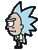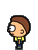There are several important formulas used in Pocket Mortys. We've listed the most useful ones below.

### XP Formula

When you defeat a Morty, the amount of XP you gain depends on the following formula:

$$XP \ Gained = \left \lfloor \frac{Defending \ Morty's \ Base \ XP \times Defending \ Morty's \ Level} {7} \times LevelModifier \times WildModifier \right \rfloor$$

See Table

###### WildModifier

1.0 for Wild Mortys, 1.5 for Trainer Mortys

###### LevelModifier
$$0.2 \leq 1.0 - 0.25 \times (Attacking \ Morty's \ Level - Defending \ Morty's \ Level) \leq 1.0$$
###### What This Means

You'll get full experience if your Morty is at or below the defending Morty's level. You will suffer a 25% XP reduction for being 1 level higher, 50% for 2, 75% for 3, and finally an 80% XP reduction for 4 or more levels higher than the defending Morty.

Note: If you used 3 Mortys to defeat a defending Morty, then the XP is split 2:1:1 (50% for the Morty that lands the final blow, 25% for the others).

### XP Per Trainer Level Formula

The amount of XP required to level up scales with your level:

$$XP \ For \ Next \ Level = \lfloor Current \ Level ^3 \rfloor$$

###### What This Means

At level 20, the amount of XP needed to reach the next level is 20 * 20 * 20 = 8,000 XP.

### Combination Formula

When you combine two Mortys, the resulting Morty's level is determined by the following formula:

$$New \ Morty's \ Level = Higher \ Level \ Morty's \ Level + \left \lfloor \frac{Lower \ Level \ Morty's \ Level}{2} \right \rfloor$$
###### What This Means

Take half of your Lower-level Morty's level (rounded down to the nearest whole number) and subtract it from the level of your higher-level Morty.

Ex: Combining a level 20 and level 15 Morty will result in a level 27 Morty. 20 + (15/2) = 20 + 7 = 27

### HP Formula

$$HP = \left \lfloor \left ( \ Base \ HP + HP \ IV + \left \lfloor \frac{ \sqrt{HP \ EV} }{4} \right \rfloor + 50 \right ) \times \frac{Morty \ Level}{50} \right \rfloor + 10$$
###### What This Means

Ex: A level 39 The One True Morty with an HP Initial Value (IV) of 5 and an HP Effort Value of 65535 would have floor((110+5+floor(ceil(sqrt(65535))/4)+50)*(39/50)+10) = 187 HP.

### HP Effort Value (EV) Formula

$$HP \ EV = \min (65535, Current \ HP \ EV \ Value + Defeated \ Morty \ Base \ HP)$$
###### What This Means

Ex: A Morty can have up to 65535 HP EV, and HP EV increases every time you defeat a wild Morty by that Morty's Base HP.

### Attack Formula

$$Attack = \left \lfloor \left ( \ Base \ Attack + Attack \ IV + \left \lfloor \frac{ \sqrt{Attack \ EV} }{4} \right \rfloor \right ) \times \frac{Morty \ Level}{50} \right \rfloor + 5$$
###### What This Means

Ex: A level 39 The One True Morty with an Attack Initial Value (IV) of 8 and an Attack Effort Value of 65535 would have floor((110+8+floor(ceil(sqrt(65535))/4))*(39/50)+5) = 146 Attack.

### Attack Effort Value (EV) Formula

$$Attack \ EV = \min (65535, Current \ Attack \ EV \ Value + Defeated \ Morty \ Base \ Attack)$$
###### What This Means

Ex: A Morty can have up to 65535 Attack EV, and Attack EV increases every time you defeat a wild Morty by that Morty's Base Attack.

### Defense Formula

$$Defense = \left \lfloor \left ( \ Base \ Defense + Defense \ IV + \left \lfloor \frac{ \sqrt{Defense \ EV} }{4} \right \rfloor \right ) \times \frac{Morty \ Level}{50} \right \rfloor + 5$$
###### What This Means

Ex: A level 39 The One True Morty with a Defense Initial Value (IV) of 5 and a Defense Effort Value of 65535 would have floor((110+5+floor(ceil(sqrt(65535))/4))*(39/50)+5) = 144 Defense.

### Defense Effort Value (EV) Formula

$$Defense \ EV = \min (65535, Current \ Defense \ EV \ Value + Defeated \ Morty \ Base \ Defense)$$
###### What This Means

Ex: A Morty can have up to 65535 Defense EV, and Defense EV increases every time you defeat a wild Morty by that Morty's Base Defense.

### Speed Formula

$$Speed = \left \lfloor \left ( \ Base \ Speed + Speed \ IV + \left \lfloor \frac{ \sqrt{Speed \ EV} }{4} \right \rfloor \right ) \times \frac{Morty \ Level}{50} \right \rfloor + 5$$
###### What This Means

Ex: A level 39 The One True Morty with a Speed Initial Value (IV) of 3 and a Speed Effort Value of 65535 would have floor((110+3+floor(ceil(sqrt(65535))/4))*(39/50)+5) = 142 Speed.

### Speed Effort Value (EV) Formula

$$Speed \ EV = \min (65535, Current \ Speed \ EV \ Value + Defeated \ Morty \ Base \ Speed)$$
###### What This Means

Ex: A Morty can have up to 65535 Speed EV, and Speed EV increases every time you defeat a wild Morty by that Morty's Base Speed.

### Disobedience Formula

$$Maximum \ Obedient \ Morty \ Level = \left \lfloor \left(\frac{41}{24}\right)\times \ Your \ Trainer \ Level + \left(\frac{199}{24} \right) \right \rceil$$ $$Minimum \ Trainer \ Level = \left \lfloor \left(\frac{24}{41}\right)\times \ Your \ Morty's \ Level + \left(\frac{199}{41} \right) \right \rceil$$
###### What This Means

If you level your Morty too far ahead of your trainer level, it will start to disobey you until you level up your trainer level. The formula gives how far ahead your Morty levels are allowed to still be obedient.

So for example, at trainer level 5, the maximum Morty level that will still obey you is 17, at trainer level 6 the maximum Morty level is 19, and so on.

### Bootcamp XP Formula

###### Level 10+ Bootcamp
$$XP \ Gain = 350 + \ rand \left( -15 \ , \ 15 \right)$$
###### Level 25+ Bootcamp
$$XP \ Gain = 2,000 + \ rand \left( -100 \ , \ 100 \right)$$
###### Level 50+ Bootcamp
$$XP \ Gain = 7,000 + \ rand \left( -300 \ , \ 300 \right)$$
###### What This Means

The Level 10+ bootcamp will give a random amount of XP between 335 and 365 XP, the level 25+ bootcamp will give a random amount of XP between 1,900 and 2,100 XP, and the level 50+ bootcamp will give a random amount of XP between 6,700 and 7,300 XP.

#### 提交评论

You are commenting as a guest. Please take the time to Register an Account if you want to keep track of your comments, set an avatar, and use more site features!